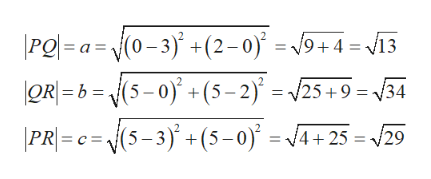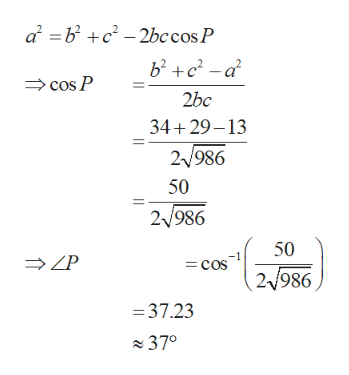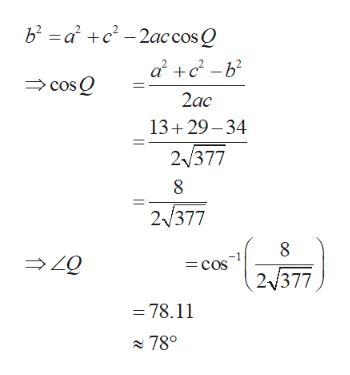# Find, correct to the nearest degree, the three angles of the triangle with the given vertices.P(3, 0),      Q(0, 2),      R(5, 5)∠RPQ =°∠PQR =°∠QRP =°

Question
33 views

Find, correct to the nearest degree, the three angles of the triangle with the given vertices.

P(3, 0),      Q(0, 2),      R(5, 5)
 ∠RPQ = ° ∠PQR = ° ∠QRP = °
check_circle

Step 1

To find the three angles of the triangle formed with the vertices P(3,0), Q(0,2), R(5,5).

Obtain the length of the sides of the triangle PQR using the distance formula as follows.help_outlineImage Transcriptionclose(0-3)' +(2-0) = v9+4 13 ORb(5-0)+(5-2) 25+9= 34 PR= c= {(5-3)+(5-0) /4+ 25 = 29 \Pg=a= fullscreen
Step 2

Find the angles using cosine rule as follows. Obtain the value of angle P as given below.help_outlineImage Transcriptionclosea bc2bccos P bca cos P 2bc 34+29-13 2/986 50 2/986 50 = cos 2/986 =37.23 370 fullscreen
Step 3

Obtain the value of angle Q ...help_outlineImage Transcriptioncloseb2 ac22accosQ ac-b cosQ 2ac 13+29-34 2/377 2/377 cos 2 377 = =78.11 780 fullscreen

### Want to see the full answer?

See Solution

#### Want to see this answer and more?

Solutions are written by subject experts who are available 24/7. Questions are typically answered within 1 hour.*

See Solution
*Response times may vary by subject and question.
Tagged in